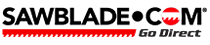Item added to cart800.754.6920
Free Ground Shipping on Orders over \$150 (except bandsaws)

How do I measure my bandsaw for you to make my blades for me?

Adjust your tension mechanism so that it is about 1/3 of the way from the closed position. Then measure the diameter of your wheels where the blade rides. Take each wheels diameter times that by 3.14 and divid by number of wheels. Then add these numbers together. Example 14? wheel1 12? wheel2 14x3.14=43.96/2=21.98 12x3.14=37.68/2=18.84 21.98+18.84=40.82

Now measure between the axles on which your wheels turn. If you have 2 wheel use this number twice. If you have more than two wheel measure from axle to axle until you return to original axle. Example 36? axle to axle so 36x2=72

Then you add your axle dimension to your wheel dimension 72+40.82=112.82?

So your blade length would be 112.82? or 9? 4 13/16?